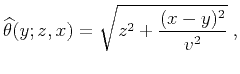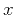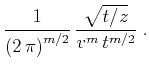Asymptotic pseudounitary stacking operatorsNext: Velocity Transform Up: EXAMPLES Previous: 3. Common-offset migration

## Post-Stack Time Migration

An interesting example of a stacking operator is the hyperbola summation used for time migration in the post-stack domain. In this case, the summation path is defined as(59)

wheredenotes the vertical traveltime,andare the horizontal coordinates on the migrated and unmigrated sections respectively, andstands for the effectively constant root-mean-square velocity (Claerbout, 1995). The summation path for the reverse transformation (demigration) is found from solving equation (59) for. It has the well-known elliptic form(60)

The Jacobian of transformingtois(61)

If the migration weighting function is defined by conventional downward continuation (Schneider, 1978), it takes the following form, which is equivalent to equation (40):(62)

The simple trigonometry of the reflected ray suggests that the cosine factor in formula (62) is equal to the simple ratio between the vertical traveltimeand the zero-offset reflected traveltime:(63)

The equivalence of the Jacobian (61) and the cosine factor (63) has important interpretations in the theory of Stolt frequency-domain migration (Levin, 1986; Chun and Jacewitz, 1981; Stolt, 1978). According to equation (22), the weighting function of the adjoint operator is the ratio of (62) and (61):(64)

We can see that the cosine factordisappears from the adjoint weighting. This is completely analogous to the known effect of dropping the Jacobian'' in Stolt migration (Harlan, 1983; Levin, 1994). The product of the weighting functions for the time migration and its asymptotic inverse is defined according to formula (10) as(65)

Thus, the asymptotic inverse of the conventional time migration has the weighting function determined from equations (10) and (62) as(66)

The weighting functions of the asymptotic pseudo-unitary operators are obtained from formulas (28) and (29). They have the form(67)(68)

The square roots of the cosine factor appearing in formulas (67) and (68) correspond to the analogous terms in the pseudo-unitary Stolt migration proposed by Harlan and Sword (1986).

Figure 1 shows the output of a simple numerical test. The synthetic zero-offset section used in this test is shown in the left plot of Figure 2. The data are taken from Claerbout (1995) and correspond to a synthetic reflectivity model, which contains several dipping layers, a fault, and an unconformity. The input zero-offset section is inverted using an iterative conjugate-gradient method and two different weighting schemes: the uniform weighting and the asymptotic pseudo-unitary weighting (67-68). I compare the iterative convergence by measuring the least-squares norm of the data residual error at different iterations. Figure 1 shows that the pseudo-unitary weighting provides a significantly faster convergence. The result of inversion after 10 conjugate-gradient iterations is shown in Figures 2 and 3. The right plot in Figure 2 shows the output of the least-squares migration. Figure 3 shows the corresponding modeled data and the residual error. The latter is very close to zero. Although this example has only a pedagogical value, it clearly demonstrates possible advantages of using asymptotic pseudo-unitary operators in least-squares migration.

migiter
Figure 1.
Comparison of convergence of the iterative least-squares migration. The dashed line corresponds to the unweighted (uniformly weighted) operator. The solid line corresponds to the asymptotic pseudo-unitary operator. The latter provides a noticeably faster convergence.migcvv
Figure 2.
Input zero-offset section (left) and the corresponding least-squares image (right) after 10 iterations of iterative inversion.migrst
Figure 3.
The modeled zero-offset (left) and the residual error (right) plotted at the same scale.Asymptotic pseudounitary stacking operatorsNext: Velocity Transform Up: EXAMPLES Previous: 3. Common-offset migration

2013-03-03